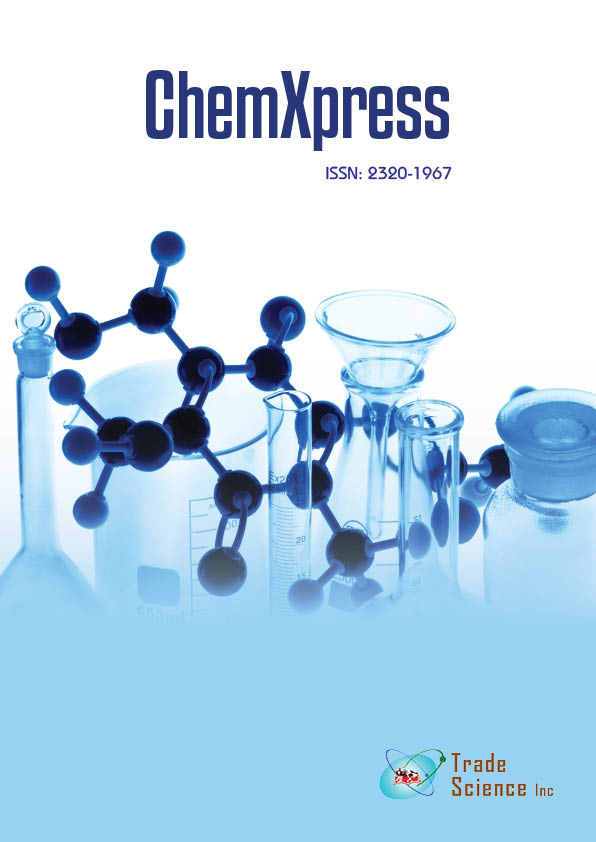Abstract

# Study of kinetics of enzymatic hydrolysis of cellulose materials

Author(s): Michael Ioelovich

In this paper, the kinetics of enzymatic hydrolysis of cellulose samples with different structural characteristics has been studied using the equation of Avrami-Kolmogarov-Erofeev (AKE): ln(1- á) = -K tn, where á is conversion degree; K is effective rate constant; t is time, and n is effective order of the kinetic process. It was shown thatAKE-equation adequately describes the experimental kinetic curves. In case of hydrolysis of highly crystalline microcrystalline cellulose, the coefficient n in the AKE-equation is 0.5, which is typical for diffusion mechanismof the process.With the decrease of crystallinity degree of cellulose, the coefficient n increases and reaches 1 for completely amorphous cellulose in awet state that indicates on the reaction of first-order. The intermediate n-value from 0.5 to 1 shows that the enzymatic hydrolysis of the sample is limited by diffusion of the large enzymemolecules into the cellulose structure. Drying of cellulose samples causes a decrease of pore volume and amplifies the contribution of diffusion to integral hydrolysis process. Effective rate constant K of enzymatic hydrolysis also increases with decreasing of crystallinity of the cellulose sample. Furthermore, the K-value for the wet sample was higher than for the dry sample. The use of parameters ofAKE-equation allows predicting the kinetics of cellulose conversion into glucose during enzymatic hydrolysis